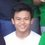# Cool integral property

Evaluate the integral: $\int_\alpha^\beta (x-\alpha)(x-\beta) dx$

SOLUTION $\int_\alpha^\beta (x^2 - (\alpha + \beta)x + \alpha\beta ) dx$

$\left(\frac{x^3}{3} - \frac{(\alpha + \beta)x^2}{2} + \alpha\beta x\right)\bigg|_{\alpha}^{\beta}$

$\frac{1}{3}(\beta^3 - \alpha^3)-\frac{\alpha+\beta}{2}(\beta^2 - \alpha^2) + \alpha\beta(\beta-\alpha)$

$\frac{1}{3}(\beta - \alpha)(\beta^2+\alpha\beta+\alpha^2)-\frac{\alpha+\beta}{2}(\beta - \alpha)(\beta + \alpha) + \alpha\beta(\beta-\alpha)$

$(\beta-\alpha)\left(\frac{1}{3}\beta^2 + \frac{1}{3}\alpha\beta + \frac{1}{3}\alpha^2 - \frac{1}{2}\alpha^2 - \alpha\beta - \frac{1}{2}\beta^2 + \alpha\beta\right)$

$(\beta-\alpha)\left(-\frac{1}{6}\beta^2 +\frac{1}{3}\alpha\beta - \frac{1}{6}\alpha^2\right)$

$(\beta-\alpha)\left[\frac{(\beta-\alpha)^2}{-6}\right]$

$\underline{\underline{-\frac{(\beta-\alpha)^3}{6}}}$

$\boxed{\int_\alpha^\beta (x-\alpha)(x-\beta) dx = -\frac{(\beta-\alpha)^3}{6}}$Note by JohnDonnie Celestre
6 years, 5 months ago

This discussion board is a place to discuss our Daily Challenges and the math and science related to those challenges. Explanations are more than just a solution — they should explain the steps and thinking strategies that you used to obtain the solution. Comments should further the discussion of math and science.

When posting on Brilliant:

• Use the emojis to react to an explanation, whether you're congratulating a job well done , or just really confused .
• Ask specific questions about the challenge or the steps in somebody's explanation. Well-posed questions can add a lot to the discussion, but posting "I don't understand!" doesn't help anyone.
• Try to contribute something new to the discussion, whether it is an extension, generalization or other idea related to the challenge.
• Stay on topic — we're all here to learn more about math and science, not to hear about your favorite get-rich-quick scheme or current world events.

MarkdownAppears as
*italics* or _italics_ italics
**bold** or __bold__ bold
- bulleted- list
• bulleted
• list
1. numbered2. list
1. numbered
2. list
Note: you must add a full line of space before and after lists for them to show up correctly
paragraph 1paragraph 2

paragraph 1

paragraph 2

[example link](https://brilliant.org)example link
> This is a quote
This is a quote
    # I indented these lines
# 4 spaces, and now they show
# up as a code block.

print "hello world"
# I indented these lines
# 4 spaces, and now they show
# up as a code block.

print "hello world"
MathAppears as
Remember to wrap math in $$ ... $$ or $ ... $ to ensure proper formatting.
2 \times 3 $2 \times 3$
2^{34} $2^{34}$
a_{i-1} $a_{i-1}$
\frac{2}{3} $\frac{2}{3}$
\sqrt{2} $\sqrt{2}$
\sum_{i=1}^3 $\sum_{i=1}^3$
\sin \theta $\sin \theta$
\boxed{123} $\boxed{123}$

## Comments

Sort by:

Top Newest

Couldn't we substitute x - average(alpha,beta) = u ?

That would have significantly reduced the computational time. Please think over it

- 6 years, 5 months ago

Log in to reply

yeah thanks.

- 5 years, 11 months ago

Log in to reply

×

Problem Loading...

Note Loading...

Set Loading...